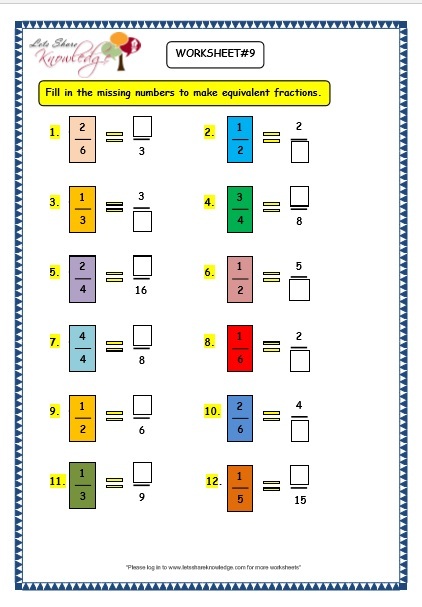Sep 29, 2016
11187 Views

# Grade 3 Maths Worksheets: (7.5 Equivalent Fractions)# Equivalent Fractions

## ExplanationExplanation of Equivalent Fractions

Worksheet 1Equivalent Fractions
Worksheet 1

Worksheet 2Equivalent Fractions
Worksheet 2

Worksheet 3Equivalent Fractions
Worksheet 3

Worksheet 4Equivalent Fractions
Worksheet 4

Worksheet 5Equivalent Fractions
Worksheet 5

Worksheet 6Equivalent Fractions
Worksheet 6

Worksheet 7Equivalent Fractions
Worksheet 7

Worksheet 8Worksheet 9Equivalent Fractions
Worksheet 9

Worksheet 10Equivalent Fractions
Worksheet 10